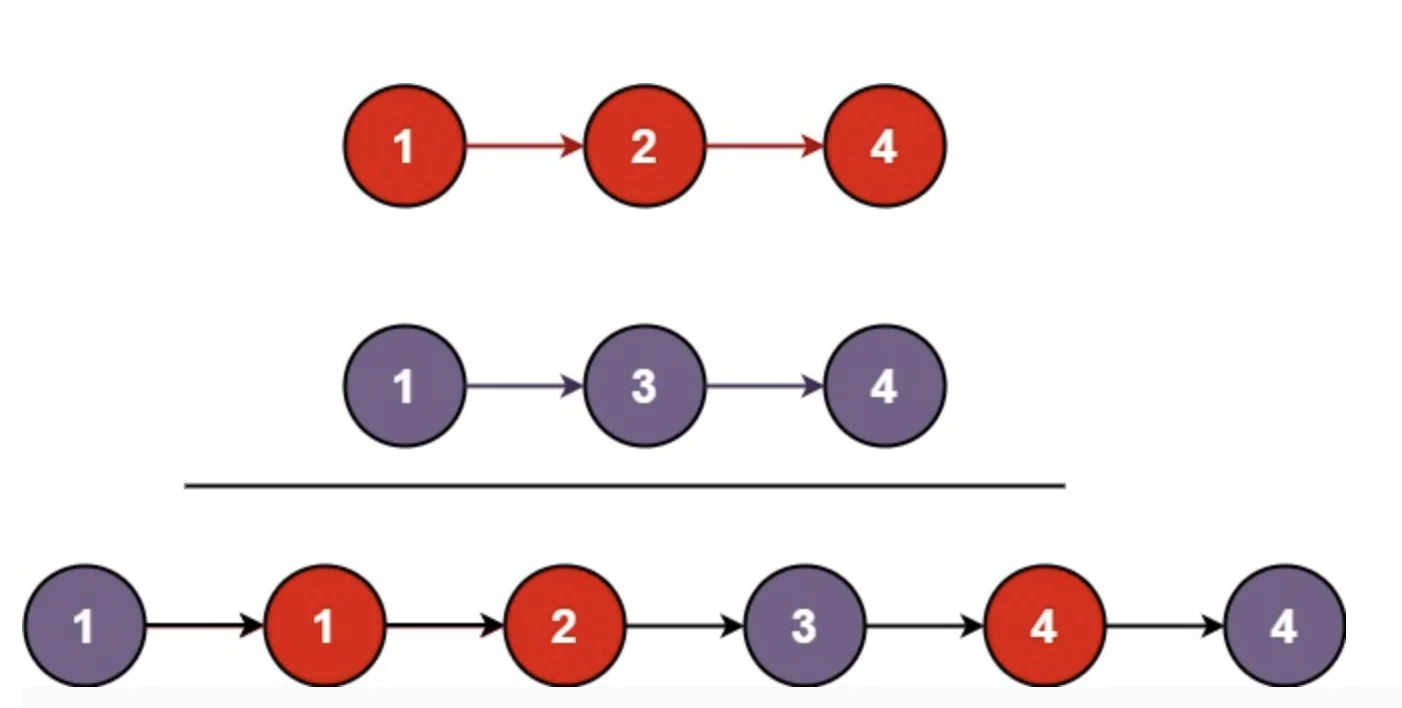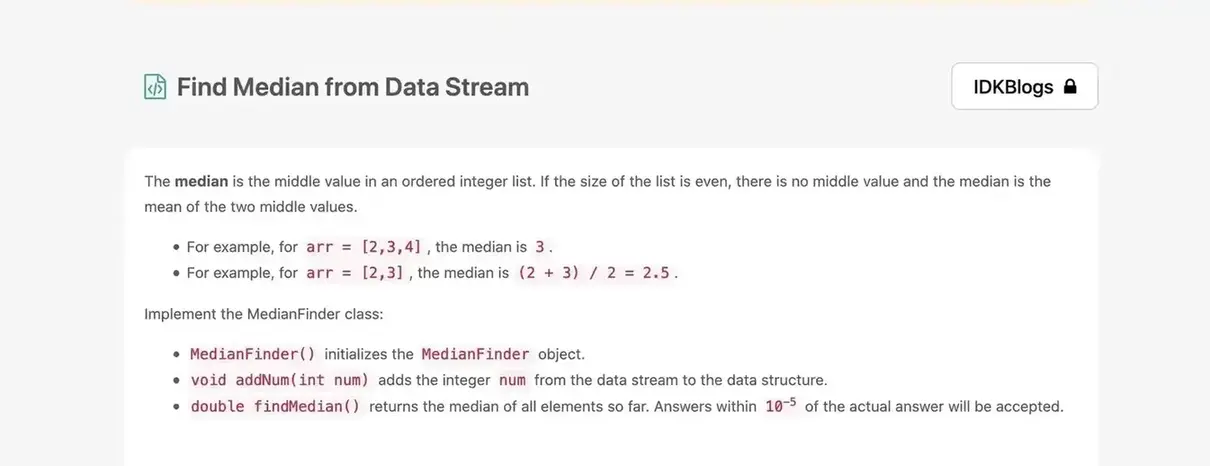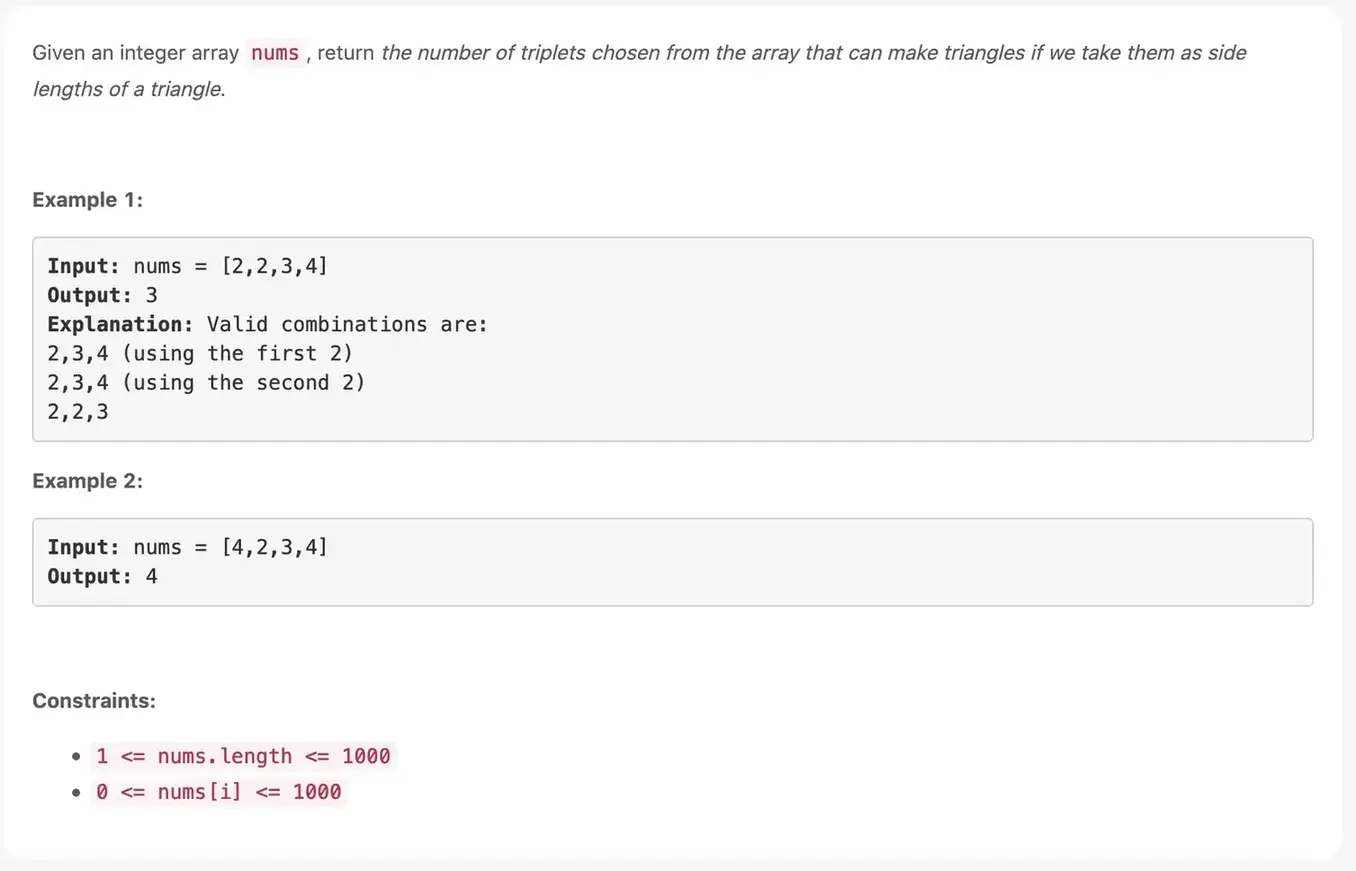# Merge Two Sorted Lists

### Merge two sorted linked lists and return it as a sorted list. The list should be made by splicing together the nodes of the first two lists.

idkblogs.com      July 11, 2021Shubham Verma# Merge Two Sorted Lists:

Merge two sorted linked lists and return it as a sorted list. The list should be made by splicing together the nodes of the first two lists.

# Example 1:

Input: l1 = [1,2,4], l2 = [1,3,4]
Output: [1,1,2,3,4,4]

# Example 2:

Input: l1 = [], l2 = []
Output: []

# Example 3:

Input: l1 = [], l2 = 
Output: 

# Constraints:

The number of nodes in both lists is in the range [0, 50].

-100 <= Node.val <=100

Both l1 and l2 are sorted in non-decreasing order.Merge Two Sorted Lists

# Solution:

``````                    /**
* @param {ListNode} l1
* @param {ListNode} l2
* @return {ListNode}
*/
var mergeTwoLists = function(l1, l2) {
var dummy = {
val : -1,
next : null
};
var curr = dummy;
while (l1 && l2) {
if (l1.val > l2.val) {
curr.next = l2;
l2 = l2.next;
} else {
curr.next = l1;
l1 = l1.next;
}
curr = curr.next;
}

curr.next = l1 || l2;

return dummy.next;

};
``````

# Output:Merge Two Sorted Lists

Thank you

To read more about the technologies, Please subscribe us, You'll get the monthly newsletter having all the published article of the last month.

### OTHER POSTS